Example 1: The Natural Base Click here to open an associated Mathcad worksheet: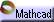Plotted below are two familiar exponential functions: y = f (x) = 2x  and  y = g (x) = 3x .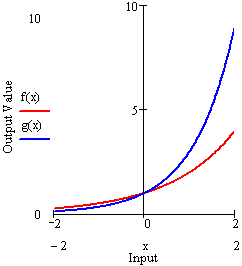On the interval shown, we see that  2x  begins with a slight head start in value, but  3x  quickly outgrows it, overtaking  2x  at  x = 0 .  We also see that the larger the values of each function, the faster they grow. Said geometrically: Both curves are steeper the further up you go.

It is interesting to compare the average growth rates of these two functions at different points. We do so in the table below. In the table, values of  f(x)  and  g(x)  have been computed at equal intervals of  Dx = 0.2 ,  beginning at  x = –2  and ending at  x = 2 . For each interval, the average rate of change  Dy/Dx  has been computed for both  y = f(x)  and  y = g(x) and these rates are displayed at the midpoint of the interval over which they have been computed.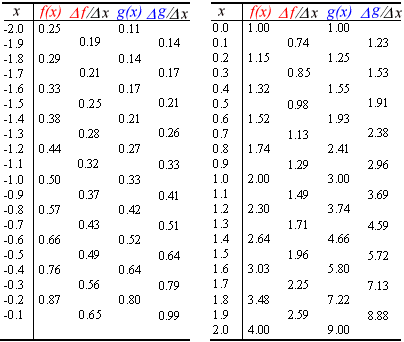It appears that the average rates of change for  f  are always lagging a little behind the values of the function itself, while those for  g  are always a little ahead. We can see this clearly if we plot each of the functions together with their computed average rates.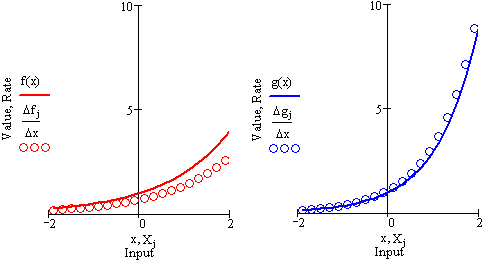Notice that, while neither curve's values coincide exactly with its growth rates, the match is a bit closer for  y = g (x) = 3x .

A reasonable question then seems to be: Is there a number, somewhere between two and three (and perhaps a bit closer to three), that is the base of an exponential function for which the growth rates are exactly the same as the values at any point? You've probably guessed the answer: The base is  e !  Below, we plot  h(x) = ex together with its average rates of change.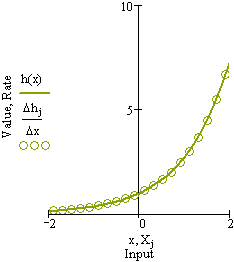The exponential function  y = ex  grows at a rate equal to its value.

This is the basic example, and the reason for  e's  preeminent place in the study of exponential and logarithmic functions.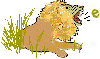The next example shows how  e  may lay hidden, like a lion in tall grass, in many numerical series.